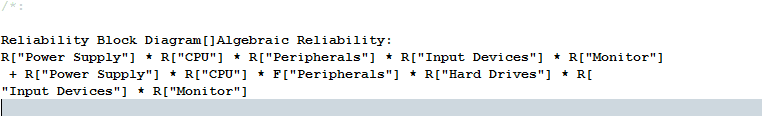Publication date: 05/24/2021

##Print Algebraic Reliability Formula

The Print Algebraic Reliability to the Log Window option prints the reliability formula for the selected system diagram to the Log window.

1. (Windows only) Select View > Log.

Or

(macOS only) Select Window > Log.

2. Open the exampleRBDcomplete.jsl file that you created.

3. In the Designs panel, select Computer.

4. Click the Computer red triangle and select Print Algebraic Reliability to the Log Window.

The Log window displays the formula for the Algebraic Reliability of the selected block diagram.

Figure 11.29 Algebraic Reliability in Log WindowNote: Reliability formulas use “R” to represent a component’s reliability and “F” to represent probability of a component’s failure.

##Generate Algebraic Expression Data Table

The Generate Algebraic Expression Data Table option creates a new data table that contains the algebraic reliability expression as a column formula. The table contains a column for each component in the system. The final column contains the system reliability algebraic expression as a JSL column formula that use the preceding columns as arguments.